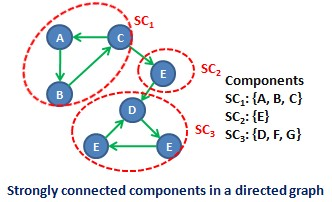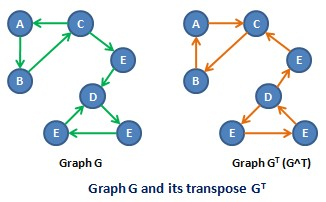## 2011年5月5日 星期四

### [ Data Structures with Java ] Section 25.2 : Strongly Connected Components

Preface :
A graphic is strongly connected if any pair of two distinct vertices v and w, there exists a path P(v,w) and a path P(w,v). In general, the vertices of a graph can be decomposed into disjoint maximum subsets of vertices that are mutually accessible; these subsets are called strongly connected (strong) component. Each component is a strong connected subgraph and the set of components is unique. Many graph algorithms begin with this decomposition; the approach often allows the original problem to be divided into subprograms, one for each strongly connected component. For instance, the graph in below figure has three strong components :Graph theory provides an assortment of algorithms for identifying strongly connected components. A simply but very inefficient O(V^2) algorithm uses repeated breadth-first searches to identify all vertices w that are reachable from a starting vertex v. The subset. The subset of such vertices w that have a path back to v is a strongly connected component. In this section, we will discuss a more efficient algorithm, which makes use of two depth-first searches. The algorithm introduces the transpose graph G^T for a Graph G. The transpose has the same set of vertices V as graph G but a new edge set E^T consisting of the edges of G but with the opposite direction. More precisely, e^T = (v, w) is in E^T if and only if e=(w,v) is in E.
Extending this fact to paths, it is clear that P^T(v,w) is a path in G^T if and only if P(w,v) is a path in G.
The algorithm follows a series of steps :
* Execute the depth-first search dfs() for the graph G, which creates the list dfsList consisting of the vertices in G in the reverse order of their finishing times.
* Generate the transpose graph G^T, the algorithm.
* Using the order of vertices in dfsList, make repeated calls to dfsVisit() for vertices in G^T. The list returned by each call is a strongly connected component of G.

Let us carry out the steps for our demonstration graph and later show why the algorithm works. Below figure includes the graph and its transpose :Begin by calling dfs() for graph G. The method returns a list of vertices ordered by visit times :
dfsList: [A, B, C, E, D ,G, F]

Using the order of vertices in dfsList, make successive calls to dfsVisit() for graph G^T :
Vertex A:
dfsVisit(A) returns the list [A, C, B] of vertices reachable from A in G^T. The set of vertices is strongly connected component SC1.
SC1={A, B, C}

Vertex E:
The vertices A, B and C have been visited. The next unvisited vertex in dfsList is E. Calling dfsVisit(E) returns the list [E]. The singleton vertex creates the strongly connected component SC2.
SC2={E}

Vertex D:
The next unvisited vertex in dfsList is D; dfsVisit(D) returns the list [D, F, G] whose elements from the last strongly connected component set SC3
SC3={D, F, G}

Why It Works :
Assume SC is the set of vertices visited by a call to dfsVisit() in G^T, in which the method uses vs as the starting vertex. Remember that vertex vs is selected as the first remaining nonvisited vertex in dfsList. We must show that SC is strongly connected; that is, for vertices v and w in SC, there exists paths P(v,w) and P(w,v) consisting of vertices in SC. We must also show that if x is a vertex in a strong component that includes vs, then x is in SC. We deal with each of these issues separately.
- Set SC Is Strongly Connected
Assume v and w are vertices in SC. They are visited by the recursive scan dfsVisit(vs), so there are paths P^T(vs, v) and P^T(vs, w) in G^T and hence paths P(v, vs) and P(w, vs). We will establish the existance of paths P(vs, v) and P(vs, w) in G. The joining of paths P(v, vs) and P(vs, w) is a path P(v, w) through vs. An argument by contradiction verifies that P(vs, v) exists. The case for (vs, w) is similar. Assume that the path P(vs, v) doesn't exist. Let us go back to the first step in the strong-component algorithm in which dfs() searches all of the vertices in G. This produces the list dfsList that defines vertices in the reverse order of their finishing times. We use the ordering to obtain the starting vertex for each dfsVisit() call in G^T. At some point, dfs() first discovers v having having color WHITE :
dfsList: [..., v, ...]

The contradiction stems from the fact that when v is first discovered, vertex vs has no valid color. Look at the different possible colors for vs.
BLACK :
Vertex vs is BLACK only if vs has already been visited by dfs() and thus vs is already in dfsList and v would have a later finishing time.
dfsList: [..., v, ..., vs, ...]

The dfsVisit() in G^T that create SC would not have chosen vs as its starting vertex. Vertex v or some previous vertex in dfsList would have been chosen. Hence, the vertex vs cannot be BLACK.

GRAY :
Some dfsVisit() in the depth-first search dfs() discovers v. If vs is GRAY, then dfsVisit() recursively scan down a path that first discovers vs. This implies a path P(vs, v), contrary to our assumption. Hence, the vertex vs cannot be GRAY.

WHITE :
Because there is a path P(v, vs), a dfsVisit() in the depth-first search will discover and subsequently visit vs before visiting v. Thus, vs will have an earlier finishing time and will occur after v in dfsList, contradicting our assumption that vs is WHITE.

Under our assumption, vertex vs would not have a valid color and so the path P(vs, v) exists. The conclusion follows that P(v, w) is a path in SC. The same argument shows that P(w, v) is a path in SC and so SC is a strongly connected component.

- SC Is a Maximal Set
To complete the algorithm, we show that if x is any vertex in a strong component that includes vs, it must be in SC. This is obvious when you recall that SC is the set of all elements visited by dfsVisit(vs) in G^T in which vs is the starting vertex. The assumption that x is in a strong component that contains vs implies that P(x, vs) exists, so P^T(vs, x) exists. Thus x would be visited by dfsVisit(vs) and hence in SC.

Implementing the strongComponents() Method :
The static method strongComponents() takes a graph g and an ArrayList as argument and implements the three steps of the strongly connected component algorithm. Initially, a call to dfs() creates dfsList. The method transpose() returns the transpose graph. The implementation of strongComponents() then uses elements in dfsList as starting vertices for repeated calls to dfsVisit() in the transpose graph. Each call to dfsVisit() for the transpose graph creates a LinkedList object which is added as an element in the ArrayList. In the end, the ArrayList, called component, is a collection of lists that represent strongly connected components in the graph. See the class JGraph for a listing of the method transpose() that return g^T as a transpose of graph g :
- Method transpose() in class JGraph :
1. public JGraph transpose() {
2.     JGraph tmpGraph = new JGraph();
3.     Iterator vtxIter = vertexTable.keySet().iterator();
5.     vtxIter = vertexTable.keySet().iterator();
6.     while(vtxIter.hasNext()) {
7.         T vtx = vtxIter.next();
8.         ArrayList> edgeList = vertexTable.get(vtx);
9.         for(Edge edge:edgeList) tmpGraph.addEdge(edge.dest, edge.source, edge.weight);
10.     }
11.     return tmpGraph;
12. }

- Method strongComponents() in class JGraph :
1. public static void strongComponents(JGraph g, ArrayList> component) {
2.     T currVertex = null;
3.     HashMap verticesColor = new HashMap();
4.     Iterator iter = g.vertexSet().iterator();
5.     while(iter.hasNext()) verticesColor.put(iter.next(), VertexColor.WHITE);
8.     dfs(g, dfsList);
9.     iter = dfsList.iterator();
10.     JGraph gt = g.transpose();
11.     while(iter.hasNext()) {
12.         currVertex = iter.next();
13.         if(verticesColor.get(currVertex).equals(VertexColor.WHITE)) {
15.             dfsVisit(gt, currVertex, dfsGTList, verticesColor, false);
16.             Collections.reverse(dfsGTList);
18.         }
19.     }
20. }

- Running Time for the strongComponents()
Recall that the depth-first search has running time O(V+E), and the computation for G^T is also O(V+E). If follows that the running time for this algorithm to compute the strong components is O(V+E).

Supplement :
* [ Data Structures with Java ] Section 25.2 : Strongly Connected Components - Example 25.2
This message was edited 9 times. Last update was at 06/04/2011 10:24:50

### [NodeJS 文章收集] NodeJS accessing file with relative path

Source From  Here Question It seemed like a straight forward problem. But I am not able to crack this. Within  helper1.js  I would like to a...